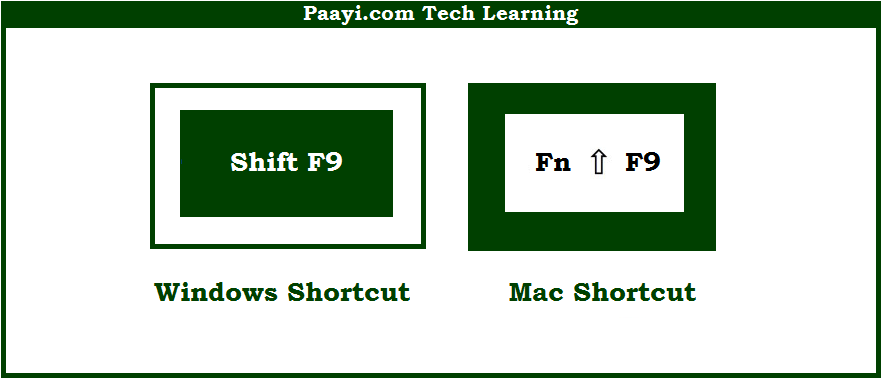# Learn How to Calculate Active Worksheet With Shortcut in Microsoft Excel

Written by | 0 Comments | 438 Views

In this article, you will learn how to Calculate Active Worksheet with a shortcut in Microsoft Excel. You will also get to know the shortcut to Calculate Active Worksheet on Mac. The easy key combinations will make you operate Microsoft Excel smoothly.

Calculate Active Worksheets With Shortcut in Microsoft Excel

Here we will show you how to calculate active worksheets in Microsoft Excel. Like many other programs Microsoft Excel also uses similar key combinations while using shortcuts.

You can use the key combinations given below to calculate the active worksheet using the shortcut. With this shortcut, you can able to calculate all the active worksheets only which are open in all the workbooks. So, here we go:

How to Calculate Active Worksheets With Shortcut in Microsoft Excel?It is used to calculate active worksheets in Microsoft Excel.  With this shortcut, you can able to calculate all the active worksheets. This way you can easily calculate active worksheets using this shortcut.